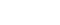## SAT Exam Syllabus

 SN SAT Exam Format 1 EBRW Section - Evidence-based reading and writinga) Reading b) Writing 2 SAT Mathematics section

## EBRW Section

The SAT Reading section comprises 52 multi-choice questions with a duration of 65 minutes. All 52 questions are derived from 5 evidence-based passages, and students/candidates are asked to analyse the information in the given passages before answering the questions. Each passage could be 500-700 words in length.

Passages’ format could be either in paragraphs or coupled with charts, tables and infographics. The passages are generally drawn from literature, social science, economics, psychology, sociology and history.

All the questions asked in the SAT Reading section fall under 3 categories:

• Factual questions are derived from the core context of the passage.
• Rhetoric questions are formulated to evaluate the grasp of tone and style conveyed in the passage.
• Synthesis questions are based on drawing a conclusion or connecting the plots between charts, passages and infographics.

## SAT Exam Format - Reading Passage Structure

All passages in the reading section are followed by 10-11 questions with 4 multi-choice answers.

• SAT Writing and Language

The SAT exam pattern of the SAT writing section comprises 44 multi-choice questions based on 4 passages and is 35 minutes in duration. When it comes to the SAT Writing section, the focus is to evaluate students’ or candidates’ ability to analyse and edit a piece of content given in the passage. Ideally, students should edit the passage, spot and rectify errors, sharpen the sentences, and correct any misinterpretation in the graphical content.

Graphs, charts and infographics accompany a few passages in the writing sections. Each passage could be closely associated to:

• Science
• Career
• History
• Humanities
• Social Studies

## The SAT exam pattern of the SAT Mathematics section comprises 58 questions in 80 minutes, where over 75% are multiple-choice types. The other 25% of questions are response types where the students must solve problems, come up with answers, and shade the appropriate numbers (grid-ins). When compared to other sections of the SAT Exam, the Math section is specially designed to assess candidates’ knowledge in math concepts and the ability to apply those to solve real-time problems.

Since students need to solve complex mathematical problems, the SAT exam pattern of the SAT Math section is categorised into two types: with a calculator and without a calculator. The below table depicts the timeframe and number of questions to be answered with and without a calculator.

 Time Allotted 80 minutes Calculator Portion (38 questions) 55 minutes No-Calculator Portion (20 questions) 25 minutes Total Questions 58 questions Multiple-Choice (4 options) 45 questions grid-in 13 questions

## The SAT Exam pattern of the Math test focuses on 4 areas :

1. Heart of Algebra
2. Problem-solving and Data Analysis

### SAT Exam Format of Heart of Algebra (19 questions)

• Analysing and fluently solving linear equations and systems of linear equations.
• Creating linear equations and inequalities to represent relationships between quantities and to solve problems.
• Understanding and using the relationship between linear equations and inequalities and their graphs to solve problems.

SAT Exam Format of Problem-solving and Data Analysis (17 questions)

• Creating and analysing relationships using ratios, proportional relationships, percentages, and units.
• Representing and analysing quantitative data.
• Finding and applying probabilities in context.

SAT Exam Format of Passport to Advanced Math (16 questions)

• Identifying and creating equivalent algebraic expressions.
• Creating, analysing, and fluently solving quadratic and other nonlinear equations.
• Creating, using, and graphing exponential, quadratic, and other nonlinear functions.

SAT Exam Format of Additional Topics in Math (6 questions)

• Solving problems related to area and volume.
• Applying definitions and theorems related to lines, angles, triangles, and circles.
• Working with right triangles, the unit circle, and trigonometric functions.
• The scores allocated to the SAT Math section are between 200-800.

Topics Tested on SAT Maths

• Miscellaneous Topics
1. Number Properties and Rounding Off
2. Order of Operations, Literal Equations and Unit Conversion
3. Literal Expressions

Concepts learned under miscellaneous topics are applied in solving questions from other topics.

6 Questions – 3 in No Calculator Section and 3 in Calculator Section

1. Line and Angle
2. Polygons - Triangle
3. Other Polygons
4. Circle
5. Solid Geometry
6. Coordinate Geometry
7. Complex Number
8. Trigonometry

• Heart of Algebra
• Questions – 8 in No Calculator Section and 11 in Calculator Section

1. Linear Equation in one variable
2. Linear Equation in two variables – Properties and Graphical Interpretation
3. System of Linear Equations
4. Linear Inequality – Properties and Graphical Interpretation
5. Absolute Value Equation and Inequality - Properties and Graphical Interpretation

16 Questions – 9 in No Calculator Section and 7 in Calculator Section

1. Laws of Indices, Radicals, Rationals, Conjugate Fractions, Long Division
2. Functions – Properties and Graphical Interpretation
3. Polynomials – Properties and Graphical Interpretation
4. Quadratic Function – Properties and Graphical Interpretation
5. Exponential Function – Properties and Graphical Interpretation
6. Sequence and Pattern Identification

• Problem Solving and Data Analysis
• Questions – All in Calculator Section

1.     Set – Venn Diagram
2.     Problems related to Percent
3.     Variation, Ratio, Time and Work, Rate and Distance
4.     Problems related to Fraction
5.     Permutation and Combination
6.     Probability
7.     Statistics
8.     Population Sampling
9.     Scatter Plots
10. Data Analysis – Graph, Chart, Table